# How to Calculate and Solve for Total Cross-Sectional Areas of Gates | Design of Gating System by a Hydraulic Method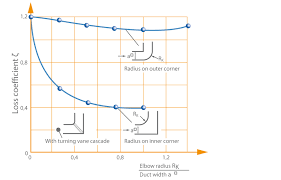The image above represents total cross-sectional areas of gates.

To compute for total cross-sectional areas of gates, four essential parameters are needed and these parameters are mass of the casting (G), density of melt (ρ), pouring time (t) and pouring speed (v).

The formula for calculating total cross-sectional areas of gates:

Fg = G / ρtv

Where:

Fg = Total Cross-Sectional Area of Gates
G = Mass of the Casting
ρ = Density of Melt
t = Pouring Time
v = Pouring Speed

Let’s solve an example;
Find the total cross-sectional areas of gates when mass of the casting is 12, density of melt is 16, pouring time is 5 and pouring speed is 25.

This implies that;

G = Mass of the Casting = 12
ρ = Density of Melt = 16
t = Pouring Time = 5
v = Pouring Speed = 25

Fg = G / ρtv
Fg = 12 / 16 x 5 x 25
Fg = 12 / 2000
Fg = 0.006

Therefore, the total cross-sectional areas of gates is 0.006 m2.

Calculating the Mass of the Casting when the Total Cross-Sectional Areas of Gates, the Density of Melt, the Pouring Time and the Pouring Speed is Given.

G = Fg x ρtv

Where;

G = Mass of the Casting
Fg = Total Cross-Sectional Area of Gates
ρ = Density of Melt
t = Pouring Time
v = Pouring Speed

Let’s solve an example;
Find the mass of the casting when the total cross-sectional areas of gates is 32, the density of melt is 4, the pouring time is 10 and the pouring speed is 2.

This implies that;

Fg = Total Cross-Sectional Area of Gates = 32
ρ = Density of Melt = 4
t = Pouring Time  = 10
v = Pouring Speed = 2

G = Fg x ρtv
G = 32 x 4 x 10 x 2
G = 2560

Therefore, the mass of the Casting is 2560 m.

Calculating the Density of Melt when the Total Cross-Sectional Areas of Gates, the Mass of the Casting, Pouring Time and the Pouring Speed is Given.

ρ = G / Fg / tv

Where;

ρ = Density of Melt
Fg = Total Cross-Sectional Area of Gates
G = Mass of the Casting
t = Pouring Time
v = Pouring Speed

Let’s solve an example;
Find the density of melt when the total cross-sectional area of gates is 15, the mass of the casting is 30, the pouring time is 8 and the pouring speed is 12.

This implies that;

Fg = Total Cross-Sectional Area of Gates = 15
G = Mass of the Casting = 30
t = Pouring Time = 8
v = Pouring Speed = 12

ρ = G / Fg / tv
ρ = 30 / 15 / 8 x 12
ρ = 2 / 96
ρ = 0.02

Therefore, the density of melt is 0.02.

Calculating the Pouring Time when the Total Cross-Sectional Areas of Gates, the Mass of the Casting, the Density of Melt and the Pouring Speed is Given.

t = G / Fg / ρv

Where;

t = Pouring Time
Fg = Total Cross-Sectional Area of Gates
G = Mass of the Casting
ρ = Density of Melt
v = Pouring Speed

Let’s solve an example;
Find the pouring time when the total cross-sectional areas of gates is 20, the mass of the casting is 14, the density of melt is 16 and the pouring speed is 10.

This implies that;

Fg = Total Cross-Sectional Area of Gates = 20
G = Mass of the Casting = 14
ρ = Density of Melt = 16
v = Pouring Speed = 10

t = G / Fg / ρv
t = 14 / 20 / 16 x 10
t = 0.7 / 160
t = 0.004375

Therefore, the pouring time is 0.004375 s.

Calculating the Pouring Speed when the Total Cross-Sectional Areas of Gates, the Mass of the Casting, the Density of Melt and the Pouring Time is Given.

v = G / Fg / ρt

Where;

v = Pouring Speed
Fg = Total Cross-Sectional Area of Gates
G = Mass of the Casting
ρ = Density of Melt
t = Pouring Time

Let’s solve an example;
Find the pouring speed when the total cross-sectional areas of gates is 7, mass of the casting is 28, density of melt is 21 and the pouring time is 2.

This implies that;

Fg = Total Cross-Sectional Area of Gates = 7
G = Mass of the Casting = 28
ρ = Density of Melt = 21
t = Pouring Time = 2

v = G / Fg / ρt
v = 28 / 7 / 21 x 2
v = 4 / 42
v = 0.095

Therefore, the pouring speed is 0.095.

Nickzom Calculator – The Calculator Encyclopedia is capable of calculating the total cross-sectional areas of gates.

To get the answer and workings of the total cross-sectional areas of gates using the Nickzom Calculator – The Calculator Encyclopedia. First, you need to obtain the app.

You can get this app via any of these means:

To get access to the professional version via web, you need to register and subscribe for NGN 1,500 per annum to have utter access to all functionalities.
You can also try the demo version via https://www.nickzom.org/calculator

Apple (Paid) – https://itunes.apple.com/us/app/nickzom-calculator/id1331162702?mt=8
Once, you have obtained the calculator encyclopedia app, proceed to the Calculator Map, then click on Materials & Metallurgical under Engineering.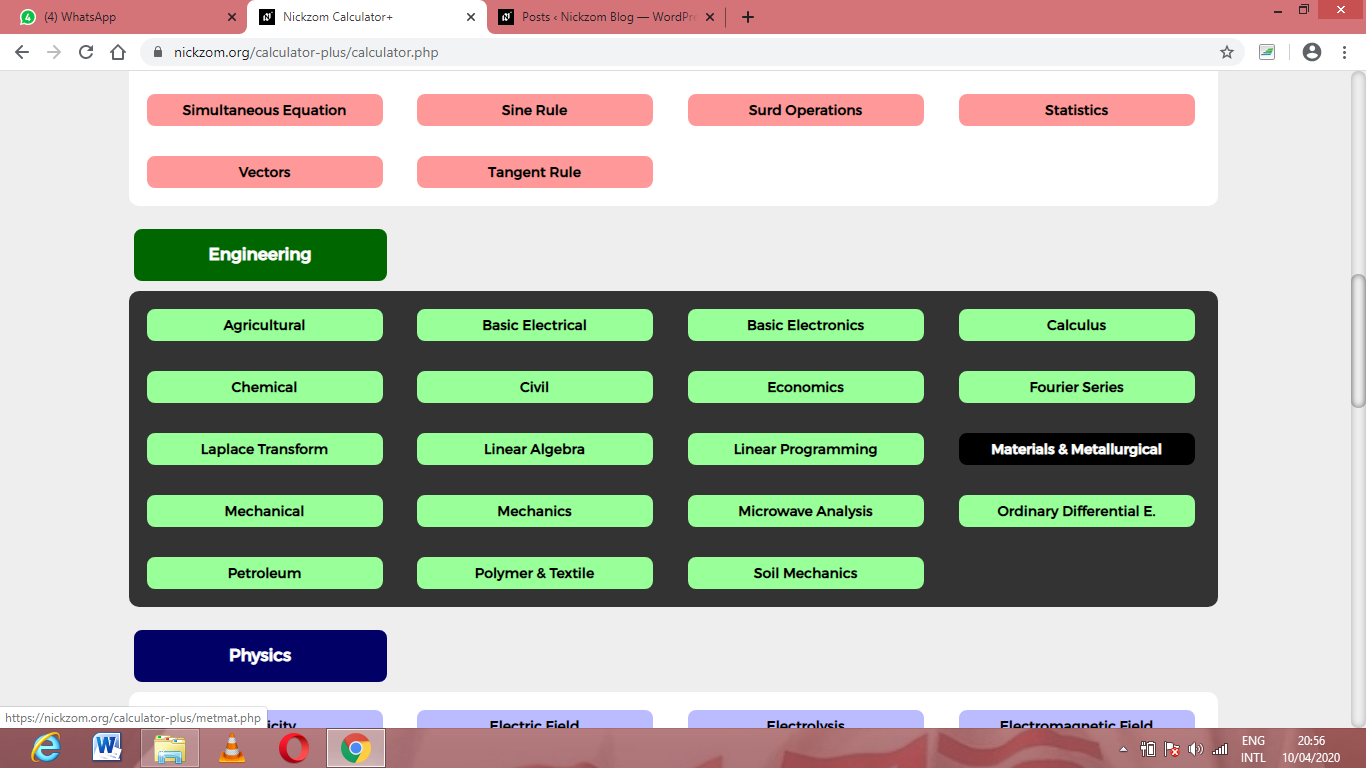Now, Click on Foundry Technology under Material & Metallurgical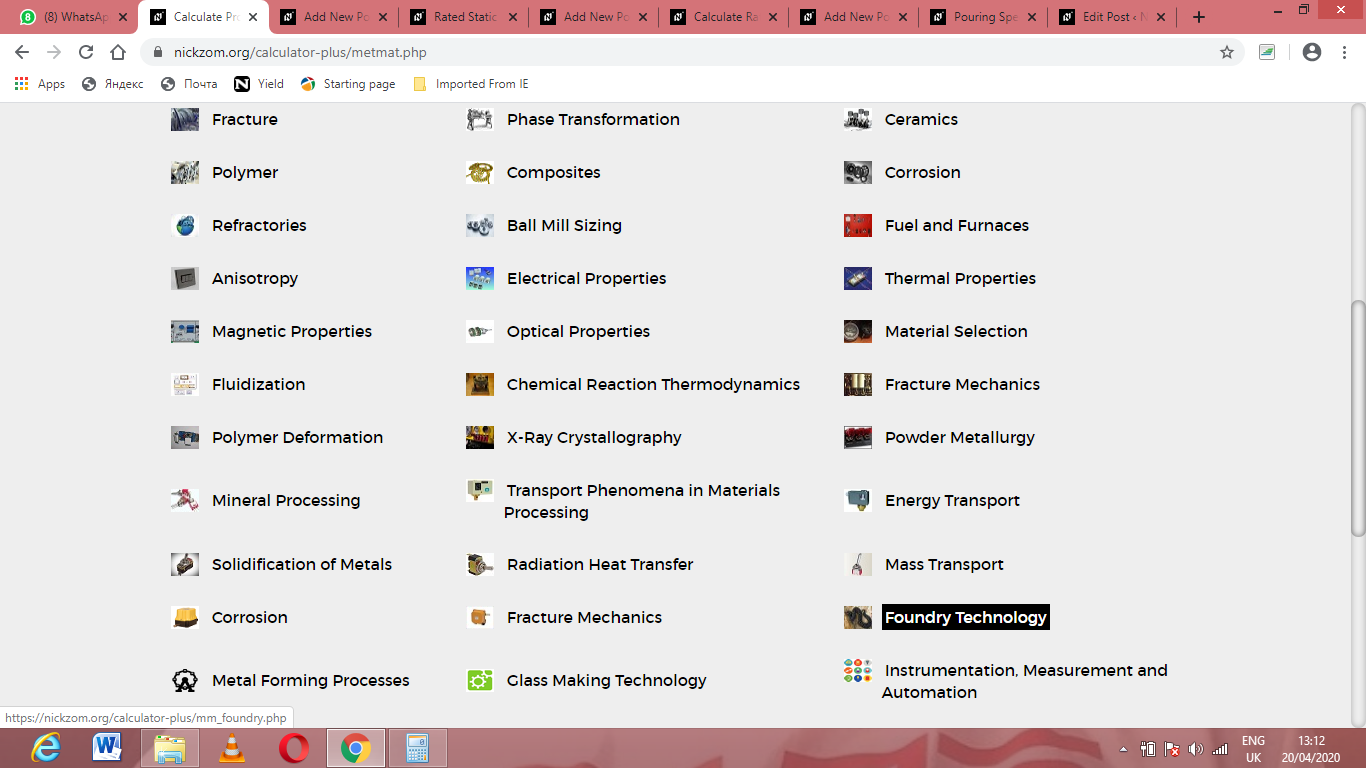Now, Click on Design of Gating System under Foundry Technology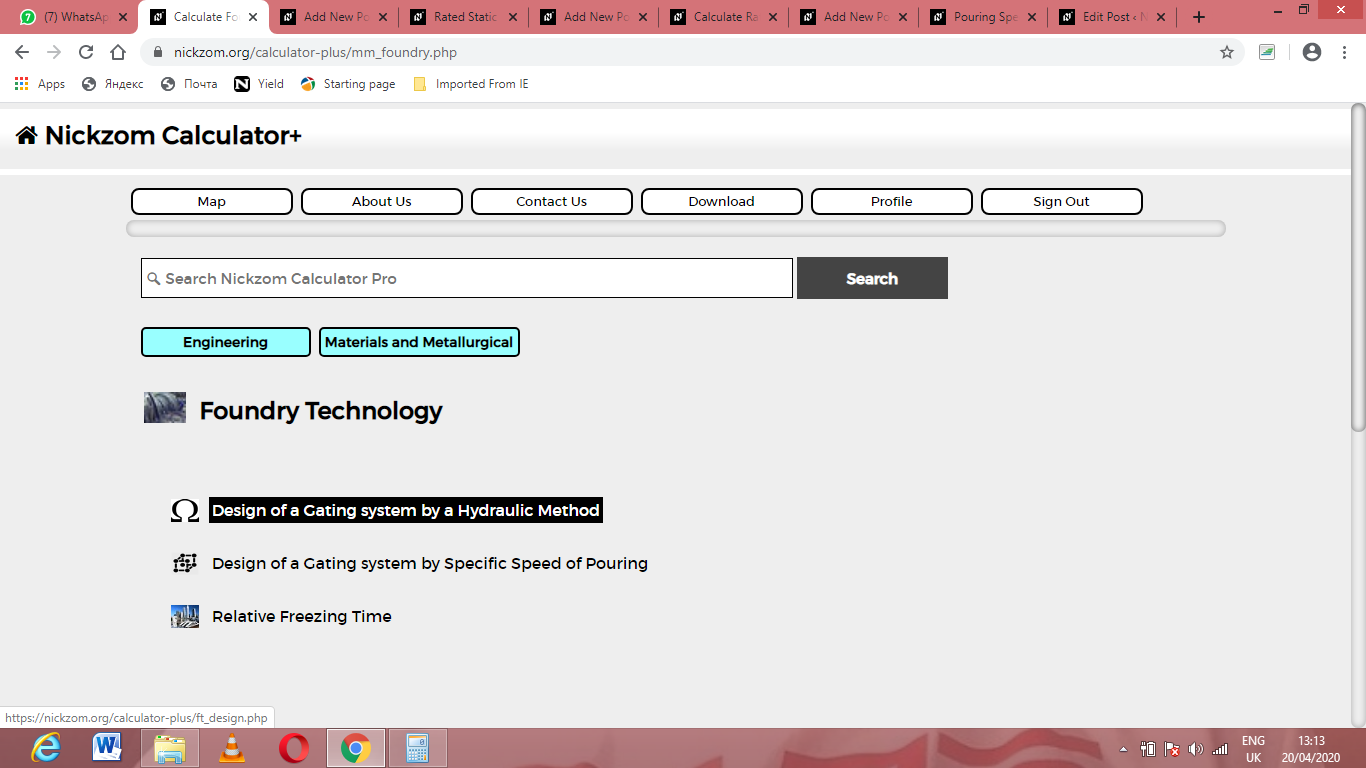Now, Click on Total Cross-Sectional Areas of Gates under Design of Gating System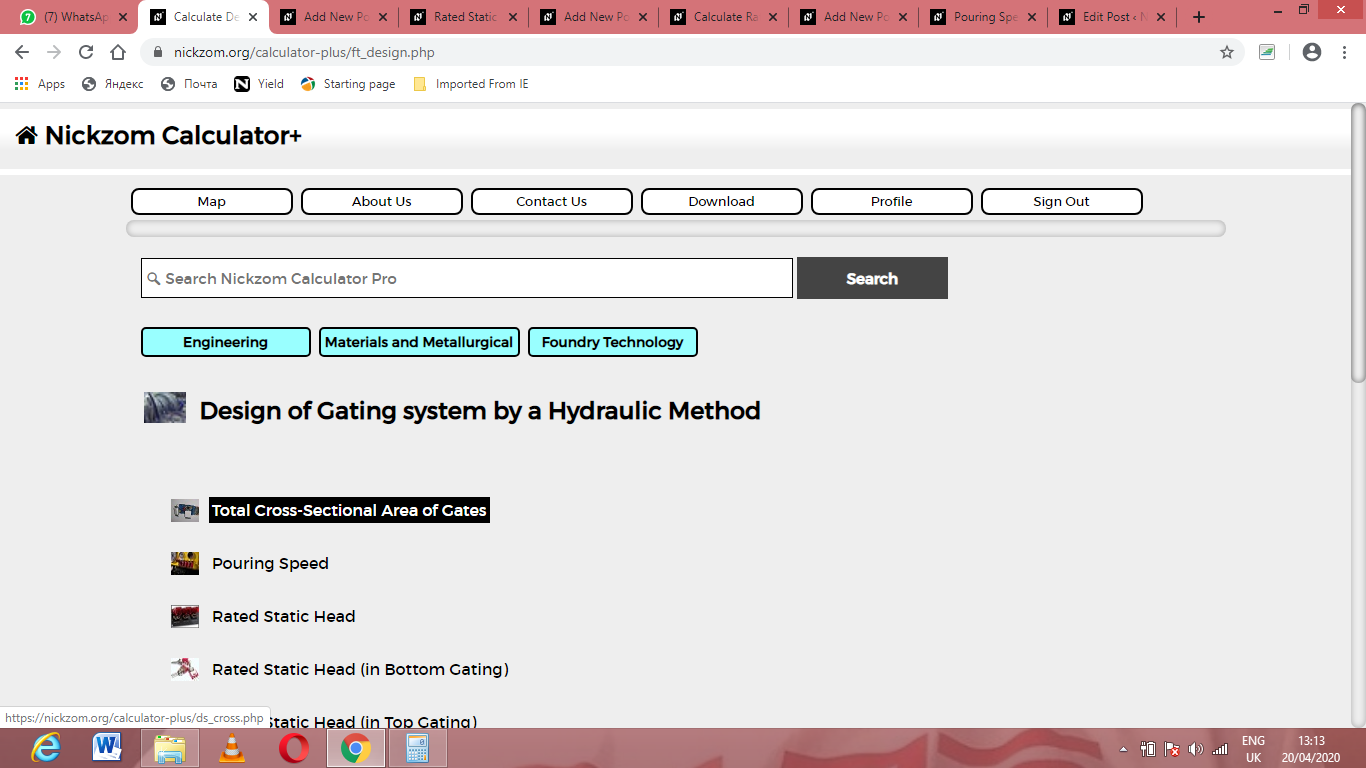The screenshot below displays the page or activity to enter your values, to get the answer for the total cross-sectional areas of gates according to the respective parameters which are the mass of the casting (G), density of melt (ρ), pouring time (t) and pouring speed (v).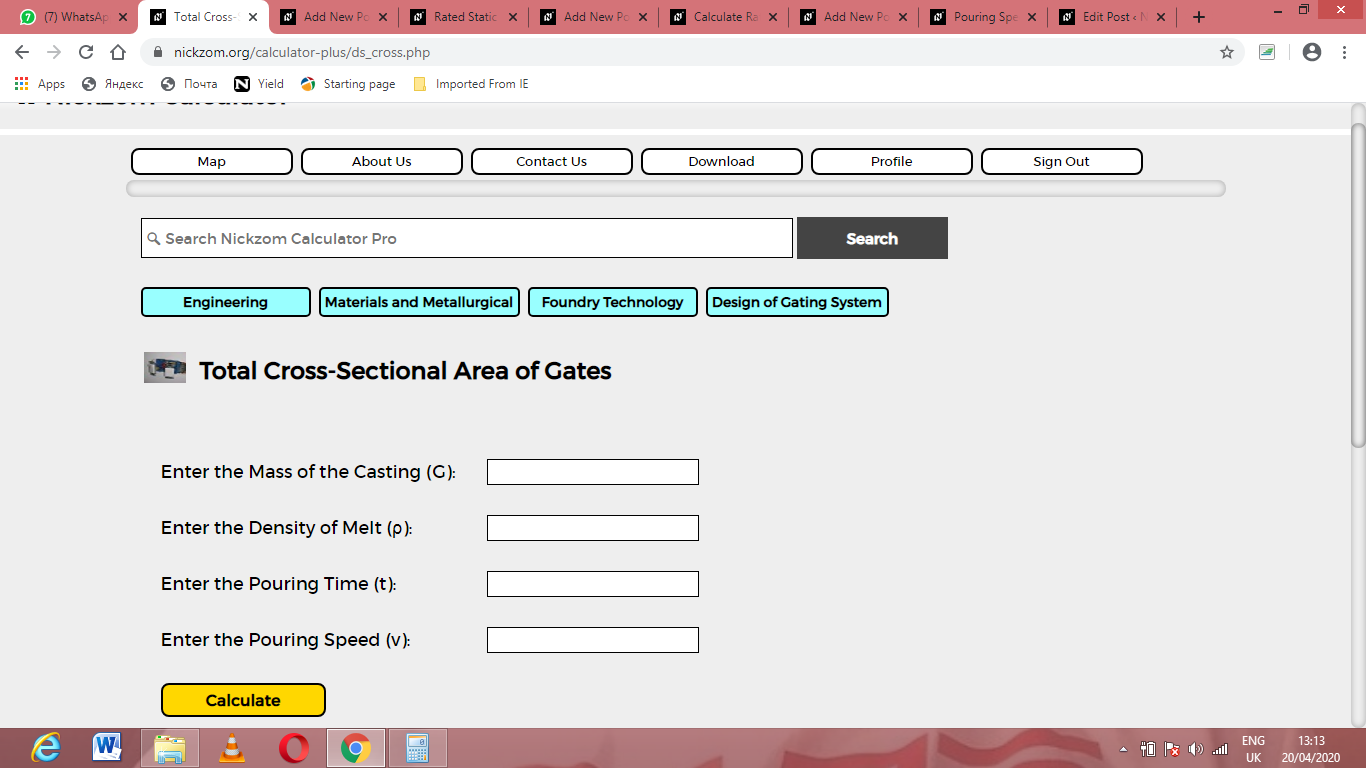Now, enter the values appropriately and accordingly for the parameters as required by the mass of the casting (G) is 12, density of melt (ρ) is 16, pouring time (t) is 5 and pouring speed (v) is 25.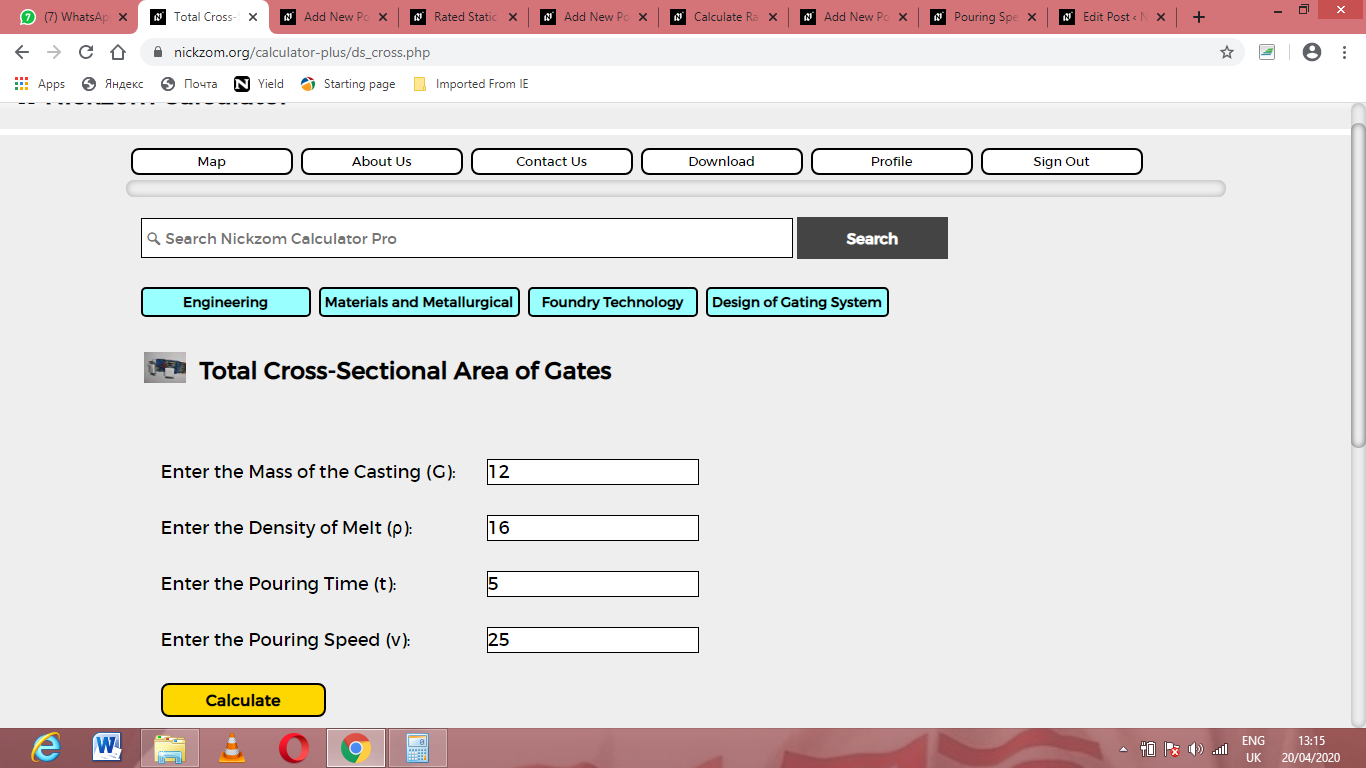Finally, Click on Calculate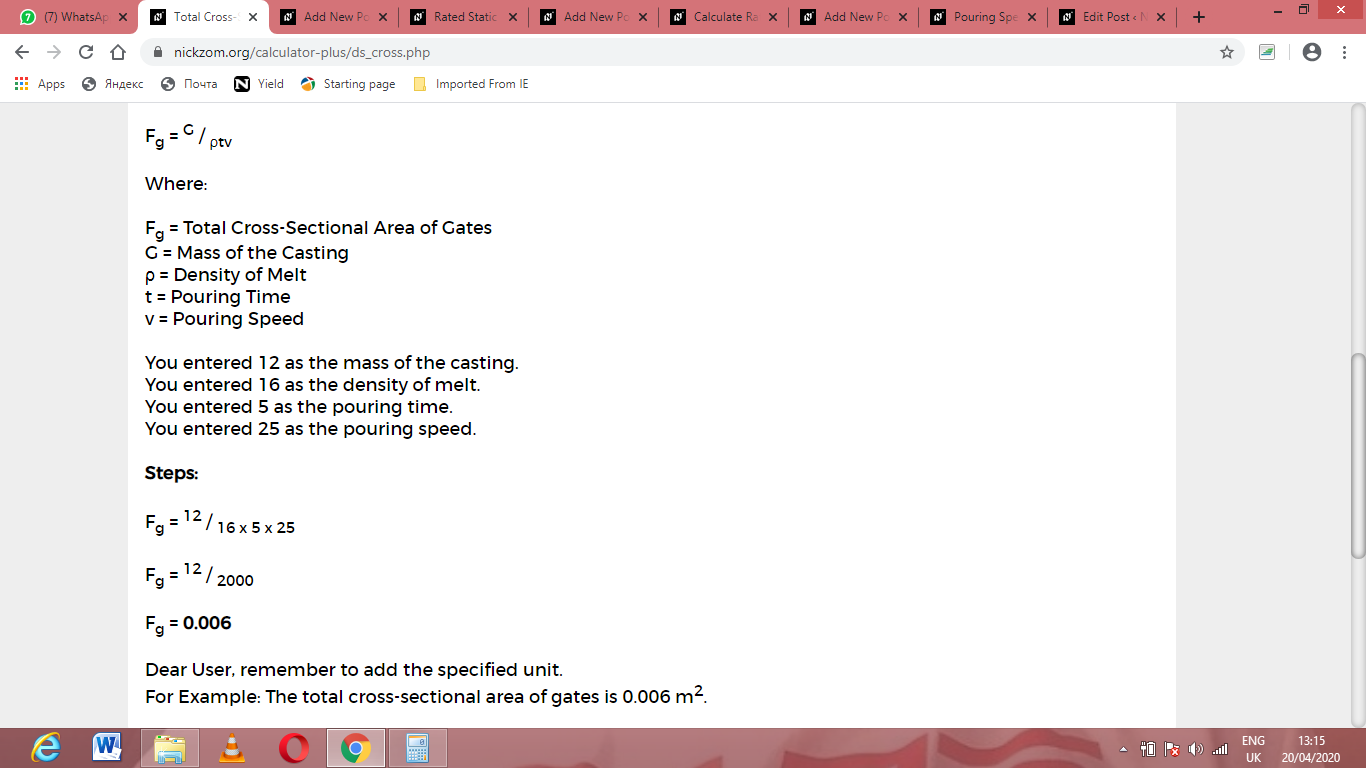As you can see from the screenshot above, Nickzom Calculator– The Calculator Encyclopedia solves for the total cross-sectional areas of gates and presents the formula, workings and steps too.